# Symmetry

 1 What is Symmetry? 2 Line of Symmetry 3 Shapes with more than One Line of Symmetry 4 Thinking out of the Box! 5 Reflection and Rotational Symmetry 6 Point Symmetry 7 Important Notes on Symmetry 8 Examples of Symmetry 9 Fun Facts on Symmetry 10 Practice Questions on Symmetry 11 Maths Olympiad Sample Papers 12 Frequently Asked Questions (FAQs) 13 FREE Worksheets on Symmetry

We at Cuemath believe that Math is a life skill. Our Math Experts focus on the “Why” behind the “What.” Students can explore from a huge range of interactive worksheets, visuals, simulations, practice tests, and more to understand a concept in depth.

Book a FREE trial class today! and experience Cuemath's LIVE Online Class with your child.

## What is Symmetry?

### Symmetry: Definition

An object has a symmetry if it can be divided into two identical pieces.

When an object has a symmetry, we say that it is symmetrical.

If an object does not have a symmetry, we say that the object is asymmetrical.

The concept of symmetry is commonly found in geometry.

The following images are symmetrical.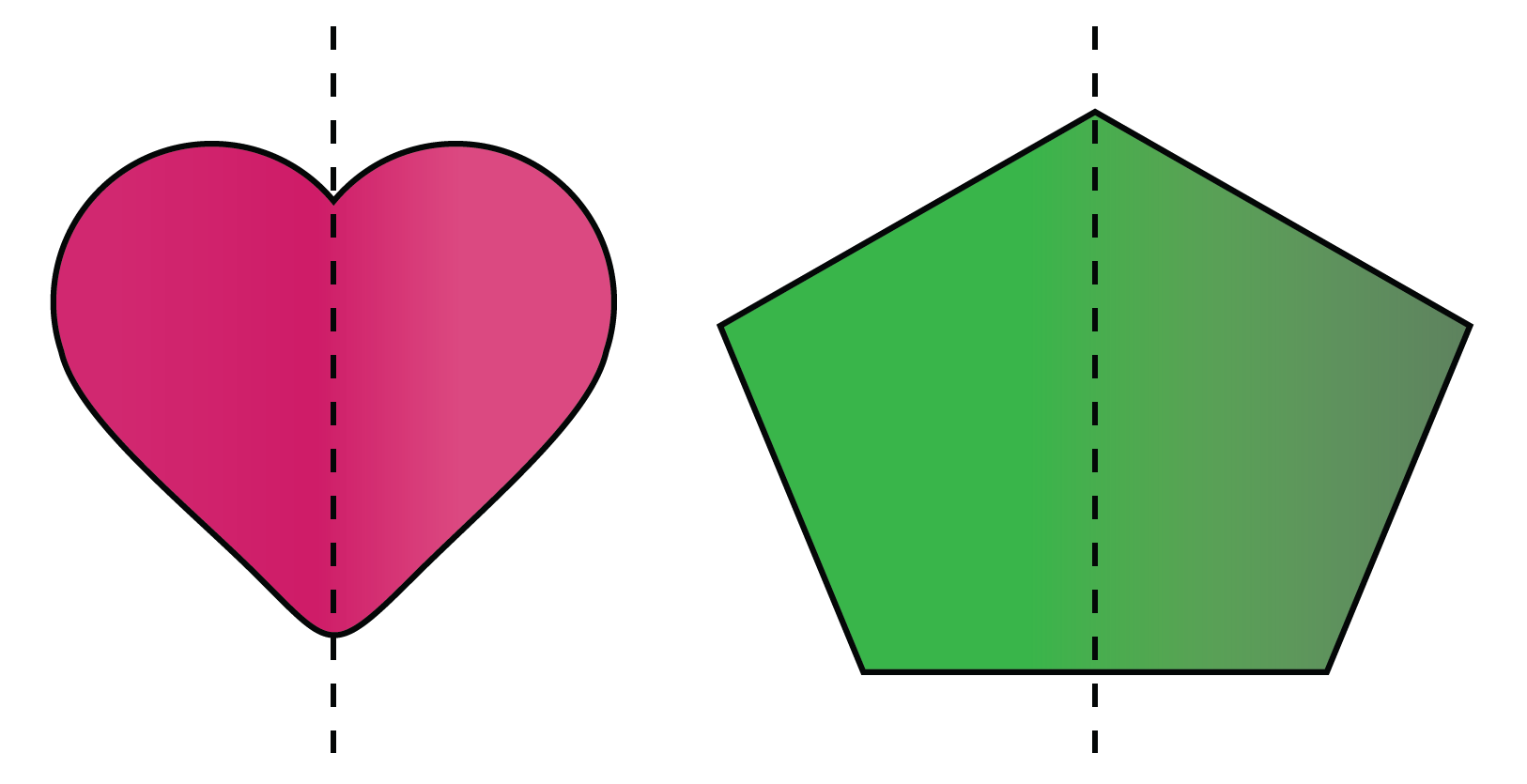## Line of Symmetry

The line of symmetry is a line that divides an object into two identical pieces.

Here, we have a star and we can fold it into two equal halves.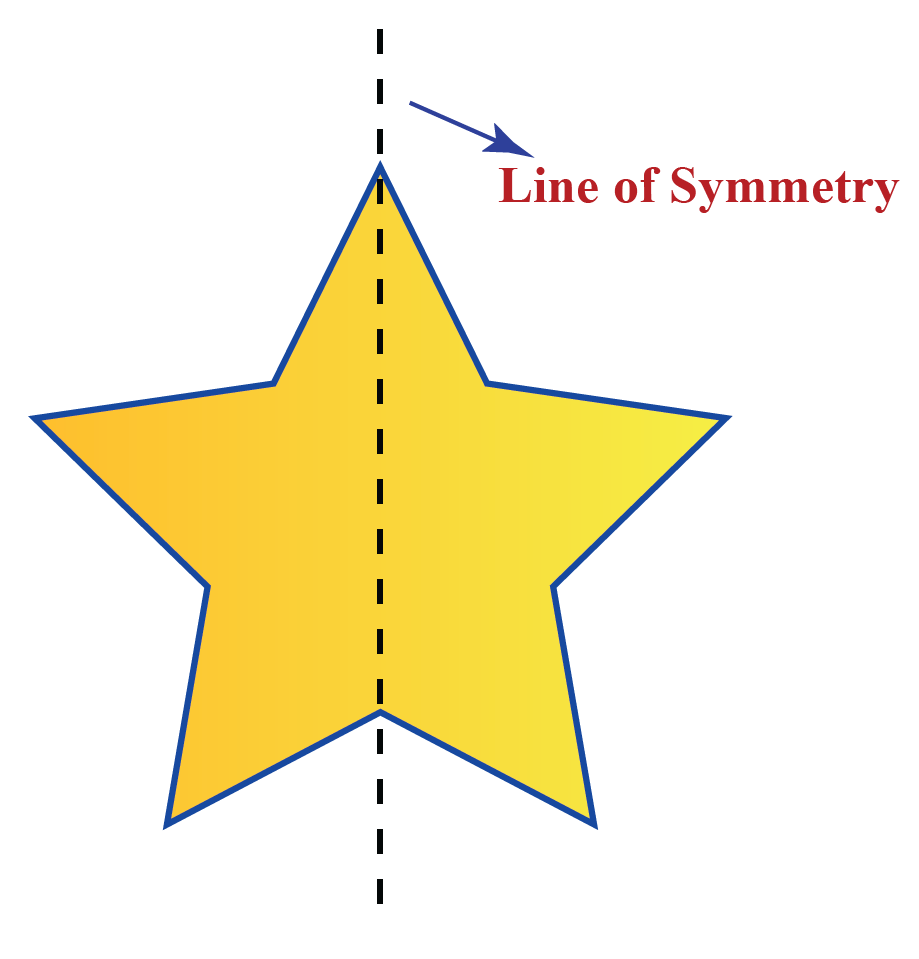If you fold a figure in half along its line of symmetry, you will notice that both the halves match each other exactly.

This line of symmetry is called the axis of symmetry.

The line of symmetry is vertical if it cuts the shape from top to bottom and vice-versa.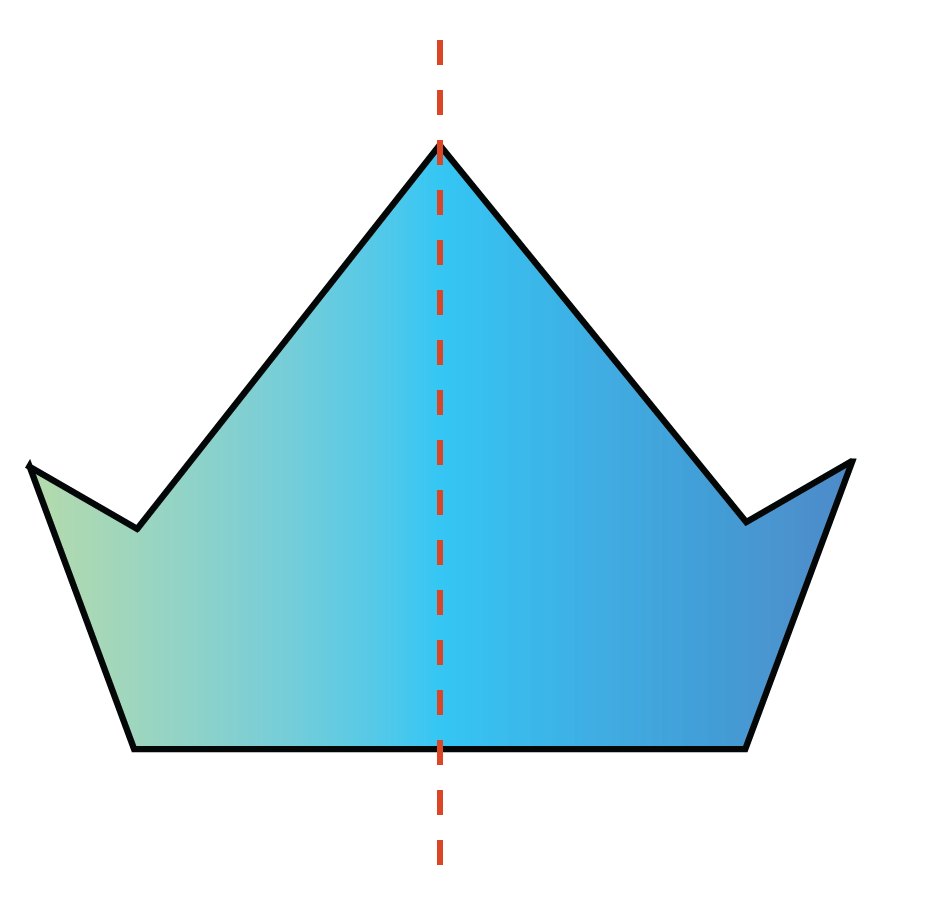The line of symmetry is horizontal if it cuts the shape from right to left and vice-versa.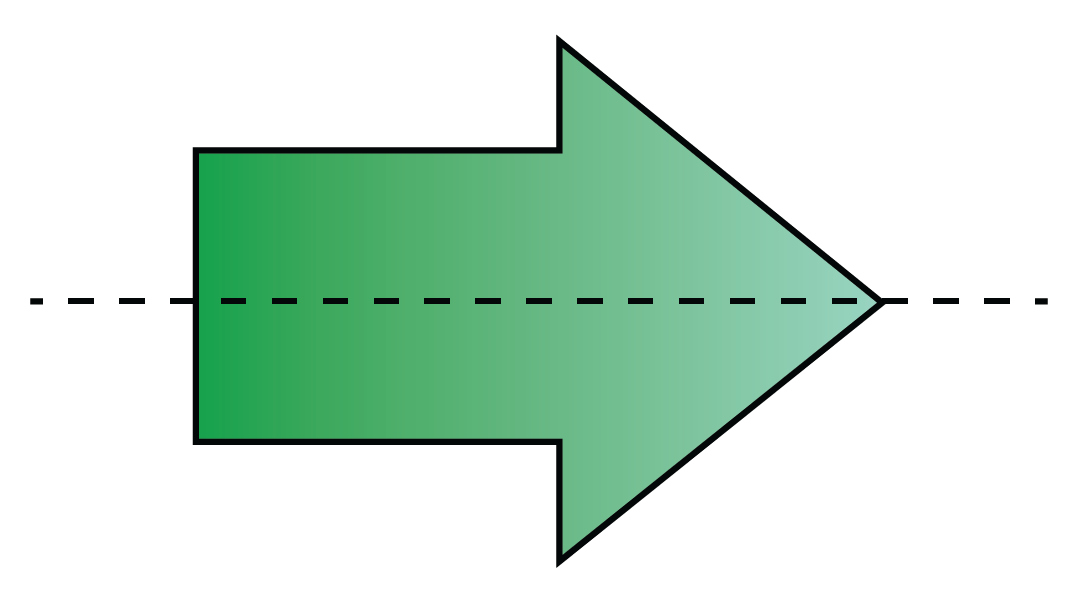Sometimes, we can split a shape across the corners to form two identical halves.

In such a case, the line of symmetry is diagonal.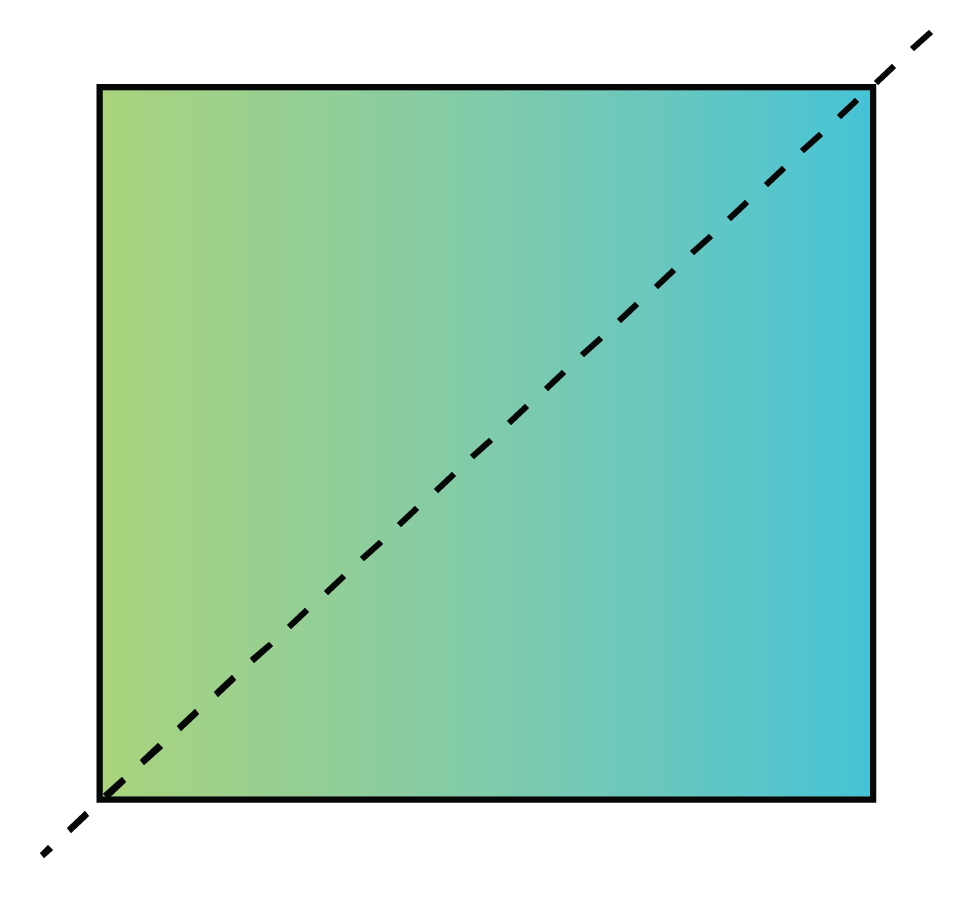Now its time to explore the symmetrical shapes visually.

Mark one line of symmetry by selecting the points

## Shapes with more than one Line of Symmetry

Some symmetrical figures have a single line of symmetry while others have more than one.

Consider this triangle.

It has only one line of symmetry.

If you try to divide it any other way, the parts will be asymmetrical.

###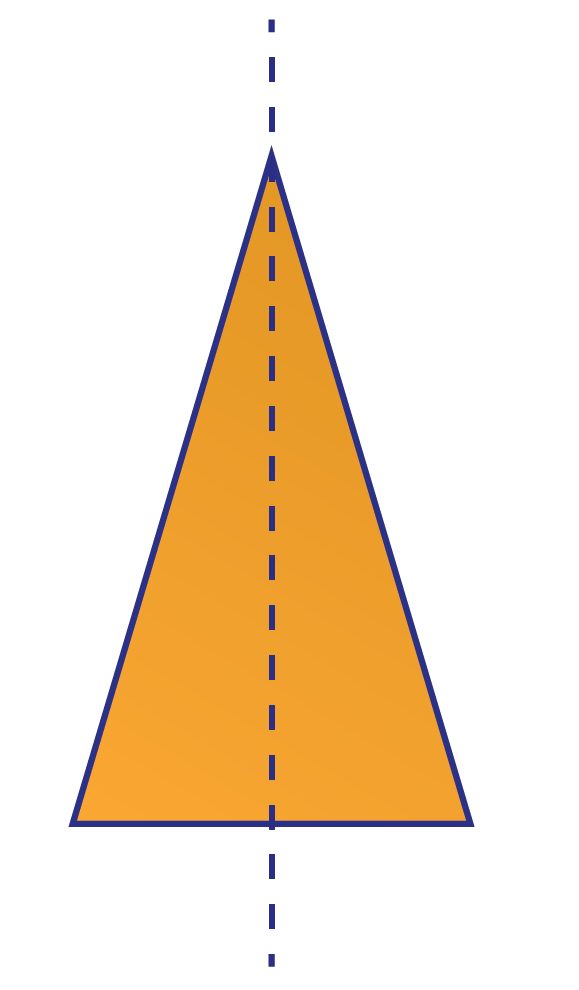In comparison to the above triangle, the one shown below has 3 lines of symmetry.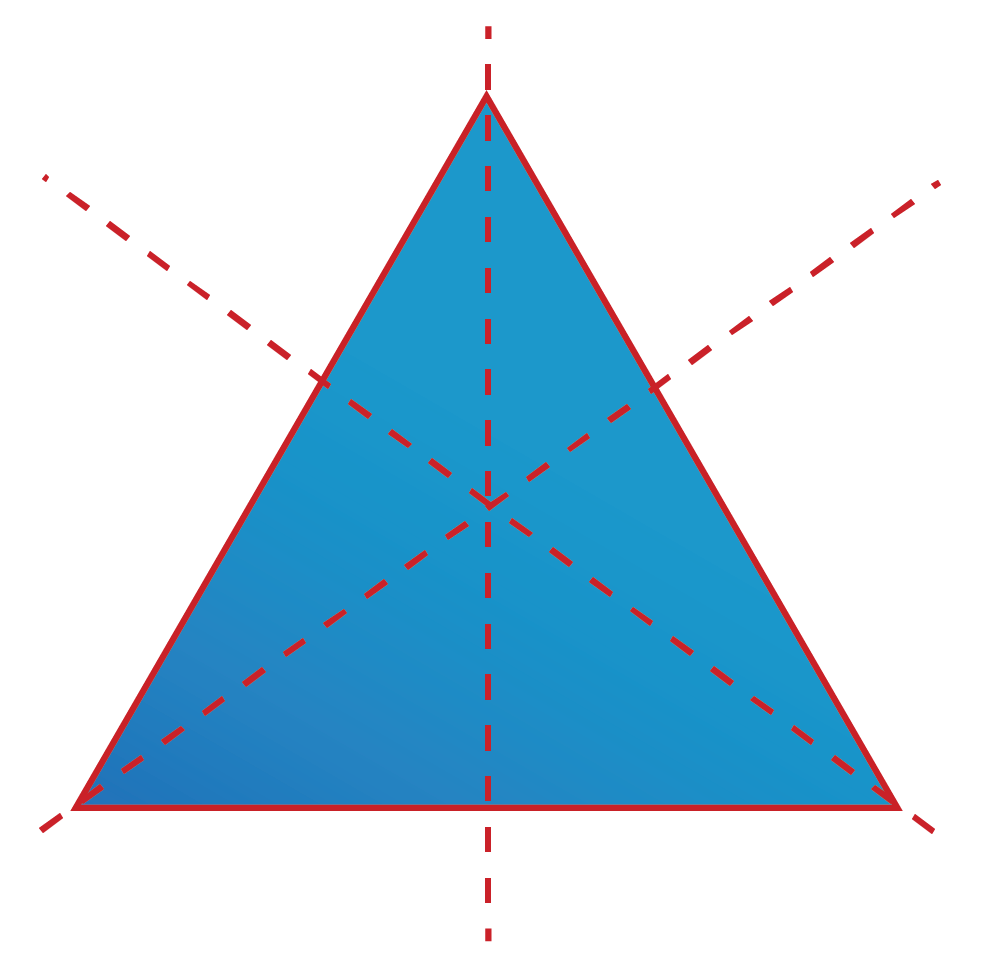Number of lines of symmetry Examples of figures
No line of symmetry Scalene triangle
Exactly one line of symmetry Isosceles triangle
Exactly two lines of symmetry Rectangle
Exactly three lines of symmetry Equilateral triangle

### Making a Symmetrical Figure: Ink String Patterns

Let us do a simple activity.

Dip short lengths of strings in different coloured paints or inks.

Now, place these strings on half of a paper.

Fold the paper and press the two halves.

Pull out the strings from the paper.

Is the resulting figure symmetric?

If yes, what is the line of symmetry?

Try out more such patterns!Think Tank

 1. The designs on the playing cards have a line of symmetry. Can you identify them for the following cards?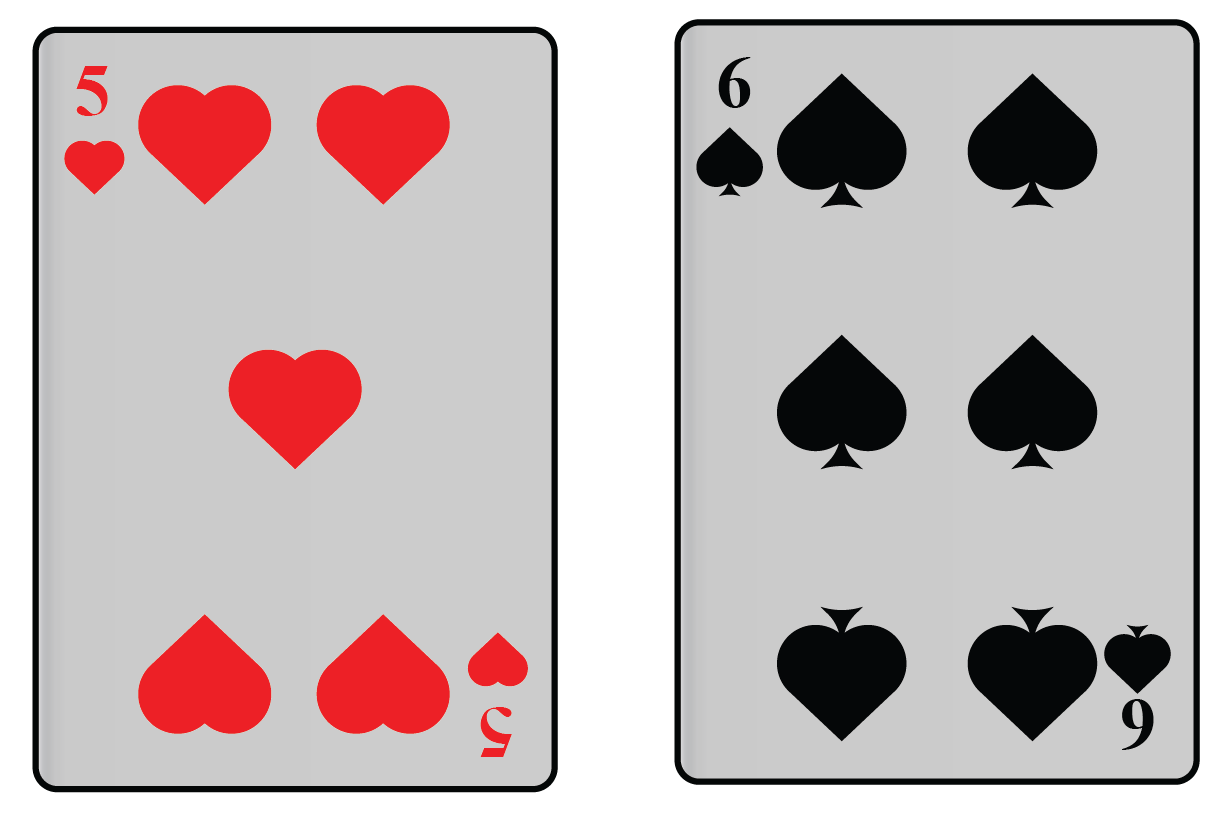2. Can you list the letters in the English alphabet from A to Z that have no lines of symmetry?

## Reflection and Rotational Symmetry

### Reflection Symmetry

When one half of an object is exactly the same as the other half of the object, it has reflection symmetry.

It can be observed that the two halves are reflections of each other.

Some human faces are identical on the left and right sides.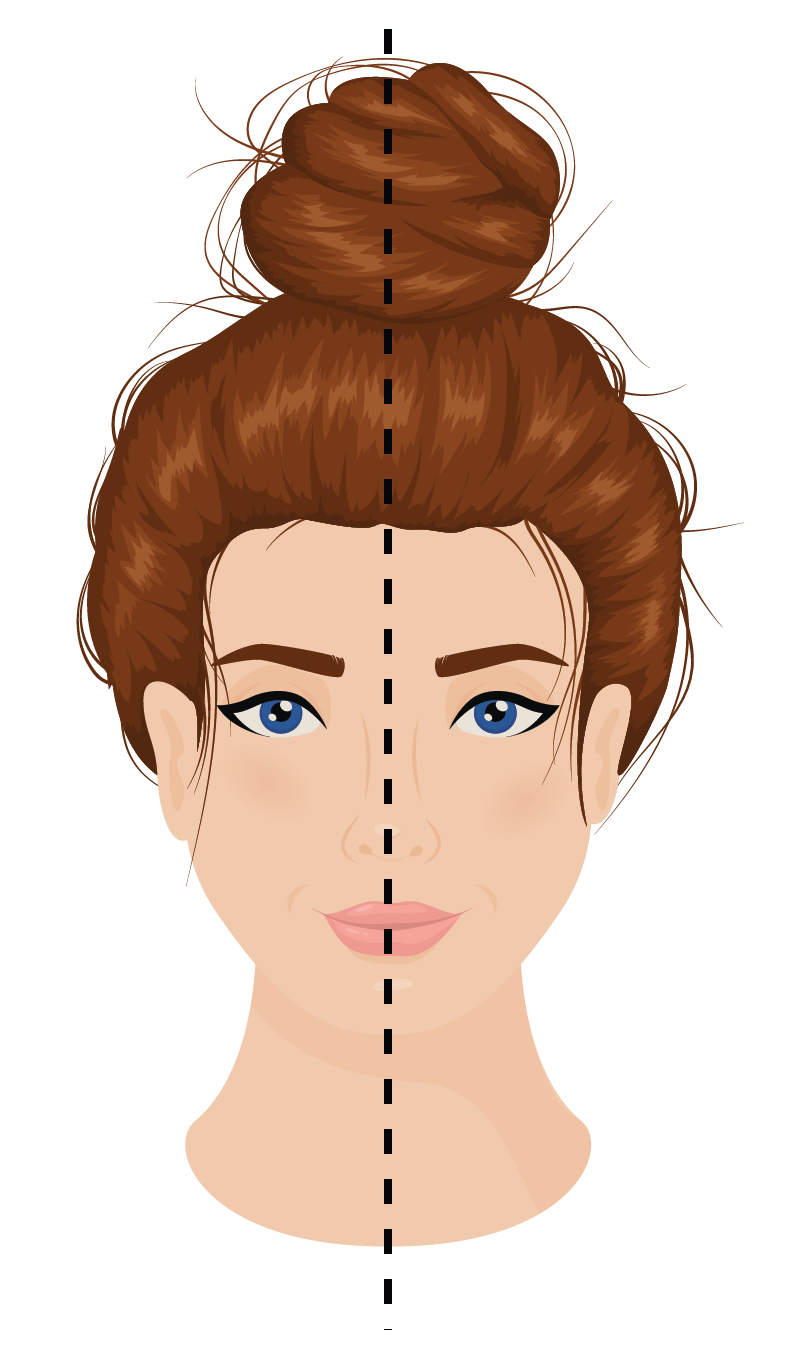Reflection of mountains and trees in clear water is an example of reflection symmetry.

### Rotational Symmetry

Look at this starfish.This starfish has 5 lines of symmetry that meet at a point P.

We can rotate the starfish about the point P.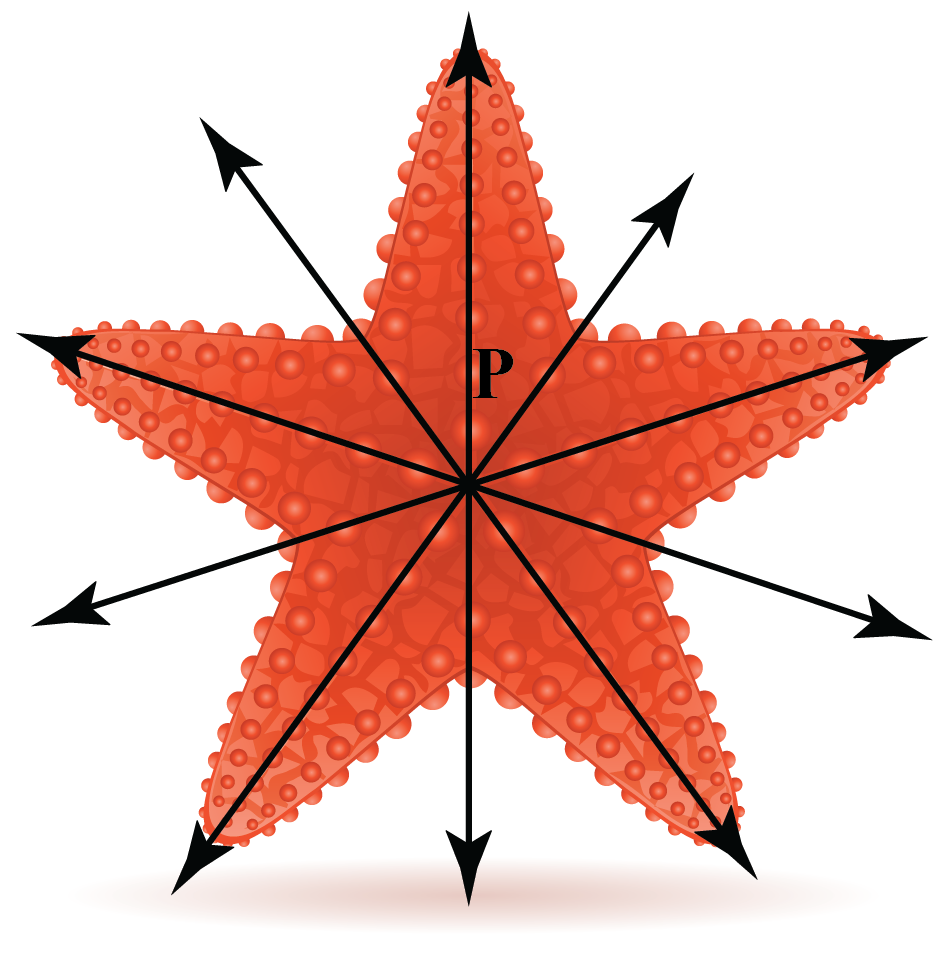If you turn or rotate the starfish about the point P, it will still look the same from all directions.

This kind of symmetry is called rotational symmetry.

The famous ferris wheel, the London Eye, is an example of rotational symmetry.

You can find many objects in real life that have rotational symmetry like wheels, windmills, road-signs, ceiling fans and so on.

Let's play with some figures having reflection and rotational symmetry.

Symmetry is everywhere around us.

Almost all plants, animals, and even humans are symmetric.

## Point Symmetry

An object has a point symmetry if every part of the object has a matching part.

Many letters of the English alphabet have a point symmetry.

The point O is the central point and the matching parts are in opposite directions.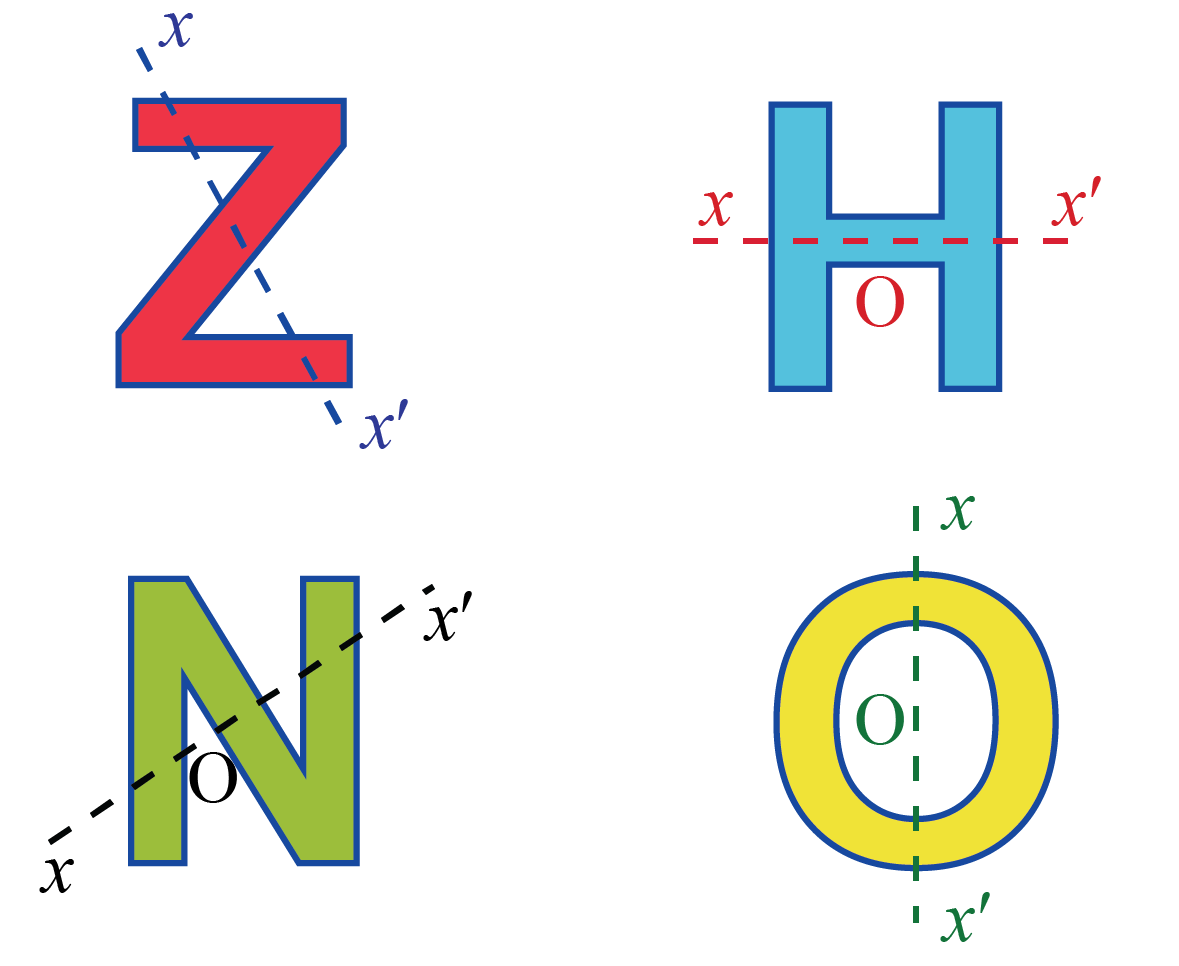If an object looks the same when you turn it upside down, then it is said to have a point symmetry.

The shape and the matching parts must be in opposite directions.

Plane and Solid Shapes
Plane and Solid Shapes
Solid shapes
Solid shapes
Polygons and Solid Shapes
Polygons and Solid Shapes
Solid Shapes and Symmetry
Solid Shapes and Symmetry
Shapes and Symmetry
Shapes and Symmetry
Solid Shapes and Symmetry